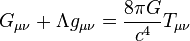# General relativity facts for kids

Kids Encyclopedia Facts
General relativity$G_{\mu \nu} + \Lambda g_{\mu \nu}= {8\pi G\over c^4} T_{\mu \nu}$
Einstein field equations

General relativity is a theory of space and time. The theory was published by Albert Einstein in 1915. The central idea of general relativity is that space and time are two aspects of spacetime. Spacetime is curved when there is gravity, matter, energy, and momentum. The links between these forces are shown in the Einstein field equations.

A central idea in general relativity is the "principle of equivalence." An example is that two people, one in an elevator sitting on the surface of the earth, and the other in an elevator in outer space accelerating at 9.8 m/s2, will each observe the same behavior of an object they drop from their hand. The object will accelerate to the floor at 9.8 m/s2 in either case, making it impossible for either to distinguish whether or not they are at rest in a gravitational field or accelerating upward at one g. Other versions of this type of "thought experiment" were used to show that light would curve in an accelerating frame of reference. There are several forms of the equivalence principle. These include: Newton's equivalence principle, the weak equivalence principle, the gravitational weak equivalence principle, Einstein's equivalence principle and the strong equivalence principle.

The Sun can be seen as this kind of valley in spacetime, and one of the other objects in the valley is the Earth. The Earth does not roll directly towards the Sun (or ball) because it is moving too fast. The force pulling the Earth towards the sun is about the same as a second force. This second force is called the centrifugal force. The centrifugal force exists because the Earth moves sideways. This sideways motion makes the distance between the Earth and Sun increase. Since the Earth is being pulled towards the sun and moving away at the same time, it stays at about the same distance. This is also how the Moon orbits the earth. In this second case, Earth is the ball and the Moon is the object.

General relativity has predicted many things which were later seen. These include:

• As light gets closer to the sun, it bends towards the sun twice as much as classical physics (the system used before general relativity) predicts. This was seen in an experiment led by Arthur Eddington in 1919. When scientists saw his experiment, they started to take general relativity seriously.
• The perihelion of the planet Mercury rotates along its orbit more than is expected under Newtonian physics. General relativity accounts for the difference between what is seen and what is expected without it.
• Redshift from gravity. When light moves away from an object with gravity (moving away from the center of the valley), it is stretched into longer wavelengths. This was confirmed by the Pound-Rebka experiment.
• The Shapiro delay. Light appears to slow down when it passes close to a massive object. This was first seen in the 1960s by space probes headed towards the planet Venus.
• Gravitational waves. They were first observed on 14 September 2015.

## Images for kidsGeneral relativity Facts for Kids. Kiddle Encyclopedia.